﻿ 液体一阶晃动模态的试验识别

# 液体一阶晃动模态的试验识别Experimental Identification of the First Sloshing Mode of Liquid

Abstract: The sloshing mode of liquid (natural frequency, mode shape and damping ratio) is the important parameter for the design of liquid-storage structure and the vibration control. In this paper, the artificial excitation is used to stimulate the first mode motion of the liquid free surface in container. The decaying free sloshing in the first mode will occur on the liquid free surface after removing the excitation. The decay curves of free vibration of wave height on the several points of liquid surface can be simultaneously measured by the several laser displacement sensors. Thus, the first natural frequency, mode shape and damping ratio of liquid sloshing can be obtained. The experimental frequencies and mode shapes agree well with theoretical results. The present experimental identification method is applicable to the arbitrary tanks.

1. 引言

2. 试验装置(a)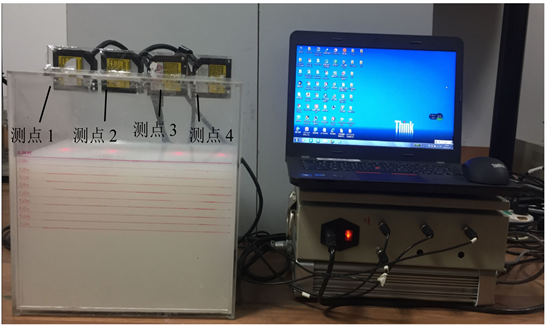(b)

Figure 1. Schematic diagram and equipment for sloshing measurement: (a) Schematic diagram for sloshing measurement; (b) Equipment for sloshing measurement

3. 自由液面波高、频率、振型与阻尼的测量

$\zeta =\frac{1}{2\text{π}\cdot \left(N-1\right)}\mathrm{ln}\left(\frac{{h}_{1}}{{h}_{N}}\right)$ (1)

4. 一阶自然频率、振型和阻尼比系数理论解Table 1. The positions of laser displacement sensorsFigure 2. Elevation drawing of rectangular tank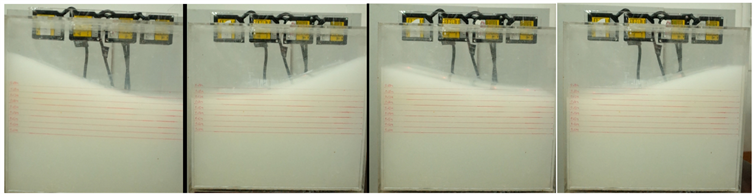Figure 3. The oscillogram of the first sloshing mode

${\omega }_{1}=\sqrt{\frac{\text{π}g}{2a}\mathrm{tanh}\left(\frac{\text{π}H}{2a}\right)}$ (2)

$Y\left(x\right)=C\mathrm{sin}\frac{\text{π}}{2a}x$ (3)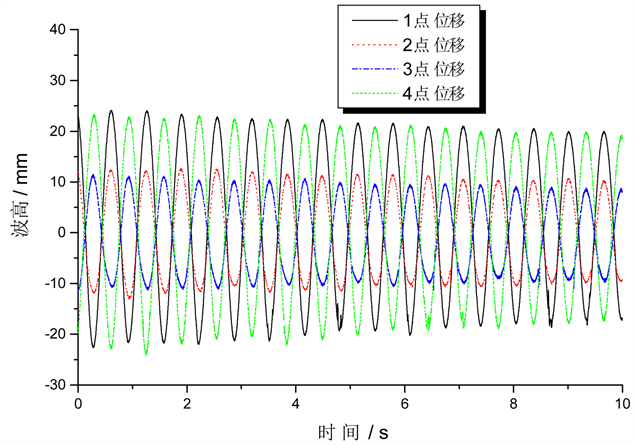Figure 4. Synchronous wave-height response curves of 4 measuring points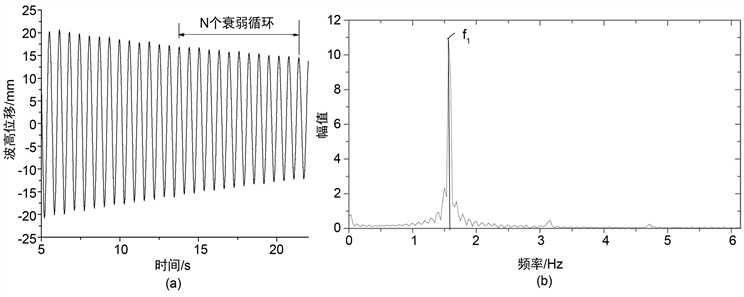Figure 5. Decay curve of free sloshing wave-height: (a) Time-response curve of wave height; (b) FFT transformation of wave height response

${\xi }_{b1}=\frac{1}{2}\sqrt{\frac{\nu }{2{\omega }_{1}}}\cdot \frac{{k}_{1}}{\mathrm{sinh}\left({k}_{1}H\right)\mathrm{cosh}\left({k}_{1}H\right)}+\frac{1}{2a}\sqrt{\frac{\nu }{2{\omega }_{1}}}\cdot \frac{\frac{1}{2}\mathrm{sinh}\left(2{k}_{1}H\right)-{k}_{1}H}{\mathrm{sinh}\left({k}_{1}H\right)\mathrm{cosh}\left({k}_{1}H\right)}+\frac{1}{2b}\sqrt{\frac{\nu }{2{\omega }_{1}}}$ (4)

${\xi }_{v1}=\frac{2\nu {k}_{1}^{2}}{{\omega }_{1}}$ (5)

${\xi }_{1}={\xi }_{b1}+{\xi }_{v1}$ (6)

5. 试验结果与理论值的比较Table 2. The first natural frequencies of liquid sloshing in a rectangular containerTable 3. The first damping ratios of liquid sloshing in a rectangular container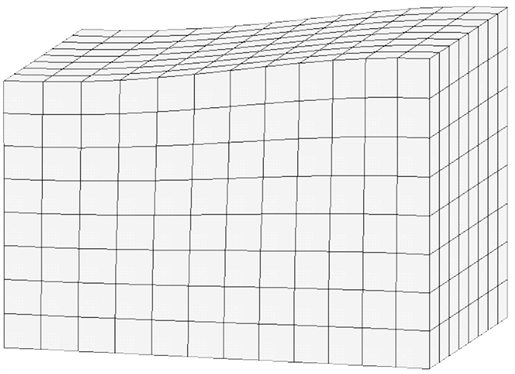Figure 6. The first sloshing mode of liquid (Ansys result)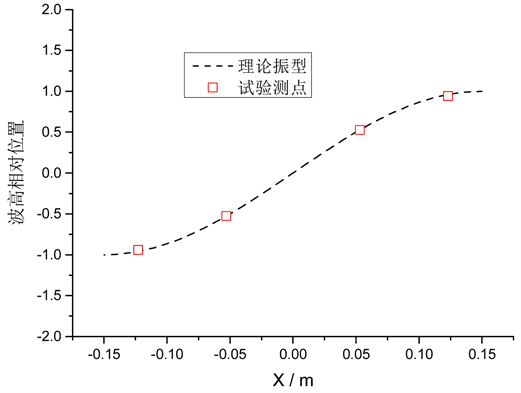Figure 7. The first sloshing mode of liquid

6. 结论

 Dodge, F.T. (2000) The New “Dynamic Behavior of Liquids in Moving Containers”. Southwest Research Institute, San Antonio, TX.

 Ibrahim, R.A. (2005) Liquid Sloshing Dynamics: Theory and Applications. Cambridge University Press, Cambridge.
https://doi.org/10.1017/CBO9780511536656

 Faltinsen, O.M. and Timokha, A.N. (2009) Sloshing. Cambridge: Cambridge University Press.

 李遇春. 液体晃动动力学基础[M]. 北京: 科学出版社, 2017.

 夏益霖. 液体晃动等效力学模型的参数识别[J]. 应用力学学报, 1991, 8(4): 27-35.

 丁文镜, 曾庆长. 晃动液体单摆模型动力学参数的频域辨识[J]. 振动工程学报, 1992, 5(3): 211-218.

 Li, Y. and Wang, Z. (2016) Unstable Characteristics of Two-Dimensional Parametric Sloshing in Vari-ous Shape Tanks: Theoretical and Experimental Analyses. Journal of Vibration and Control, 22, 4025-4046.
https://doi.org/10.1177/1077546315570716

 王立时, 李遇春, 张皓. 二维晃动自然频率与阻尼比系数的试验识别[J]. 振动与冲击, 2016, 35(8): 173-176.

 Clough, R.W. and Penzien, J. (2003) Dynamics of Structures. 3rd Edition, Computers & Structures, Inc., Berkeley, CA.

Top